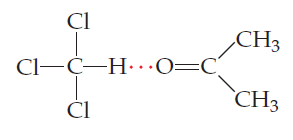# Problem: At 35 oC the vapor pressure of acetone, (CH3 )2 CO, is 361 torr, and that of chloroform, CHCl3, is 301 torr. Acetone and chloroform can form weak hydrogen bonds between one another; the chlorines on the carbon give the carbon a sufficient partial positive charge to enable this behavior:A solution composed of an equal number of moles of acetone and chloroform has a vapor pressure of 250 torr at 35 oC.What would be the vapor pressure of the solution if it exhibited ideal behavior?

###### FREE Expert Solution

We’re asked to determine the vapor pressure of an ideal solution composed of an equal number of moles of acetone and chloroform given the vapor pressure of each pure component

The given solution is composed of chloroformCHCl3 and acetone,CH3COCH3 which are both volatile

For a solution that exhibits ideal behavior with volatile solute and solvent, Raoult’s Law is given as:

$\overline{){{\mathbf{P}}}_{{\mathbf{solution}}}{\mathbf{=}}\mathbf{\left(}\mathbf{P}{\mathbf{°}}_{{\mathbf{CHCl}}_{\mathbf{3}}}{\mathbf{\chi }}_{{\mathbf{CHCl}}_{\mathbf{3}}}\mathbf{\right)}{\mathbf{+}}{\mathbf{\left(}}{\mathbf{P}}{{\mathbf{°}}}_{{\mathbf{CH}}_{\mathbf{3}}{\mathbf{COCH}}_{\mathbf{3}}}{{\mathbf{\chi }}}_{{\mathbf{CH}}_{\mathbf{3}}{\mathbf{COCH}}_{\mathbf{3}}}{\mathbf{\right)}}}$

where P˚ = vapor pressure of pure component and χ = mole fraction of component

Recall that the mole fractions in a solution add up to 1. Since the solution is composed of equal amounts of chloroform and acetone, this means:

99% (452 ratings)###### Problem Details

At 35 oC the vapor pressure of acetone, (CH3 )2 CO, is 361 torr, and that of chloroform, CHCl3, is 301 torr. Acetone and chloroform can form weak hydrogen bonds between one another; the chlorines on the carbon give the carbon a sufficient partial positive charge to enable this behavior:A solution composed of an equal number of moles of acetone and chloroform has a vapor pressure of 250 torr at 35 oC.

What would be the vapor pressure of the solution if it exhibited ideal behavior?